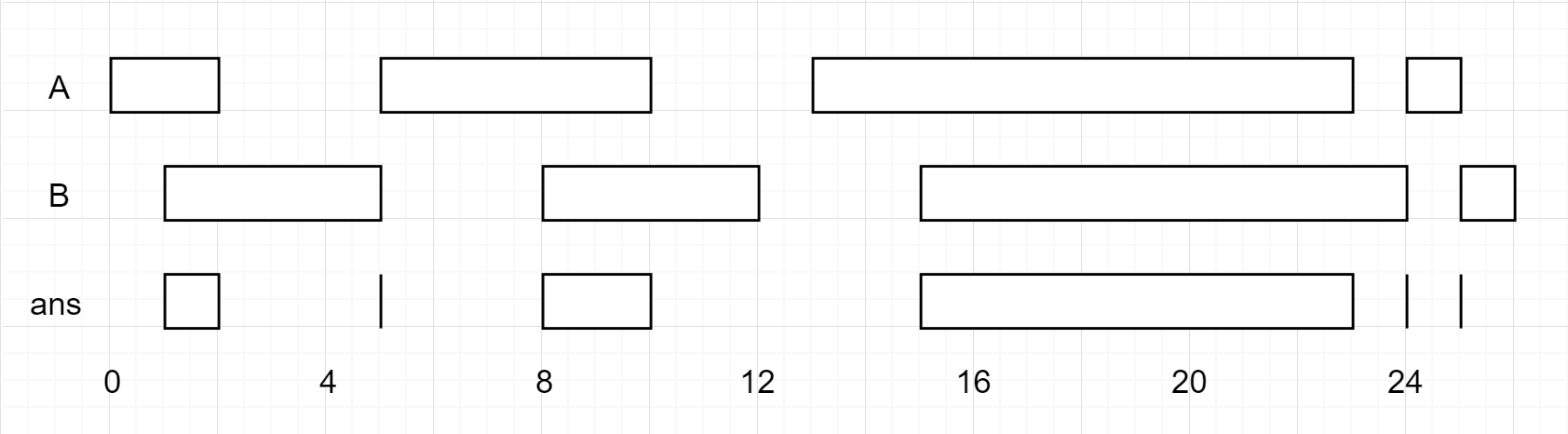Formatted question description: https://leetcode.ca/all/986.html

# 986. Interval List Intersections

Medium

## Description

Given two lists of closed intervals, each list of intervals is pairwise disjoint and in sorted order.

Return the intersection of these two interval lists.

(Formally, a closed interval [a, b] (with a <= b) denotes the set of real numbers x with a <= x <= b. The intersection of two closed intervals is a set of real numbers that is either empty, or can be represented as a closed interval. For example, the intersection of [1, 3] and [2, 4] is [2, 3].)

Example 1:Input: A = [[0,2],[5,10],[13,23],[24,25]], B = [[1,5],[8,12],[15,24],[25,26]]

Output: [[1,2],[5,5],[8,10],[15,23],[24,24],[25,25]]

Note:

1. 0 <= A.length < 1000
2. 0 <= B.length < 1000
3. 0 <= A[i].start, A[i].end, B[i].start, B[i].end < 10^9

## Solution

Since the two arrays A and B are already sorted, loop over the two arrays of intervals to obtain the intersections.

Use a list to store the intersections. Initialize index1 and index2 to be both 0. While index1 < A.length and index2 < B.length, obtain interval1 = A[index1] and interval2 = B[index2] and calculate the intersection of interval1 and interval2. If the intersection is not empty, add the intersection to the list. Then move index1 or index2 or both indices forward by 1 step. If an interval has a smaller end value, move the corresponding index forward by 1 step. If both intervals have the same end value, move both indices forward by 1 step.

After all the intersections are obtained, use a 2D array to store the intersections and return.

Java

• class Solution {
public int[][] intervalIntersection(int[][] A, int[][] B) {
List<int[]> intersectionList = new ArrayList<int[]>();
int length1 = A.length, length2 = B.length;
int index1 = 0, index2 = 0;
while (index1 < length1 && index2 < length2) {
int[] interval1 = A[index1];
int[] interval2 = B[index2];
int intersectionLow = Math.max(interval1, interval2);
int intersectionHigh = Math.min(interval1, interval2);
if (intersectionLow <= intersectionHigh) {
int[] curIntersection = {intersectionLow, intersectionHigh};
}
int high1 = interval1, high2 = interval2;
if (high1 <= high2)
index1++;
if (high1 >= high2)
index2++;
}
int size = intersectionList.size();
int[][] intersectionArray = new int[size];
for (int i = 0; i < size; i++) {
int[] curIntersection = intersectionList.get(i);
intersectionArray[i] = curIntersection;
intersectionArray[i] = curIntersection;
}
return intersectionArray;
}
}

• // OJ: https://leetcode.com/problems/interval-list-intersections/
// Time: O(M+N)
// Space: O(1)
class Solution {
public:
vector<vector<int>> intervalIntersection(vector<vector<int>>& A, vector<vector<int>>& B) {
int M = A.size(), N = B.size();
vector<vector<int>> ans;
for (int i = 0, j = 0; i < M && j < N; ) {
int s = max(A[i], B[j]), e = min(A[i], B[j]);
if (s <= e) ans.push_back({ s, e });
if (A[i] < B[j]) ++i;
else ++j;
}
return ans;
}
};

• # 986. Interval List Intersections
# https://leetcode.com/problems/interval-list-intersections/

class Solution:
def intervalIntersection(self, firstList: List[List[int]], secondList: List[List[int]]) -> List[List[int]]:
i = j = 0
n, m = len(firstList), len(secondList)
res = []

while i < n and j < m:
s1, e1 = firstList[i]
s2, e2 = secondList[j]

s, e = max(s1, s2), min(e1, e2)

if s <= e:
res.append([s, e])

if e1 <= e2:
i += 1
else:
j += 1

return res